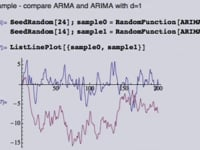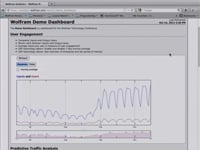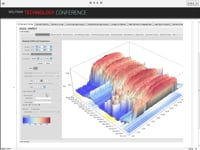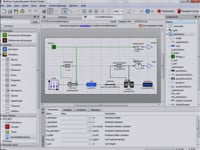Learn how »

# Numerical Differential Equations

Mathematica provides a natural interface to algorithms for numerically solving differential equations. In this presentation from the Wolfram Technology Conference, Rob Knapp gives an overview of the interface and the types of equations that can be solved, with an emphasis on features new to Mathematica 9.Reliability Analysis17:08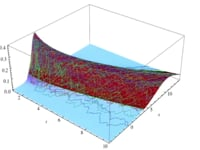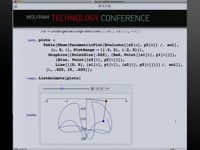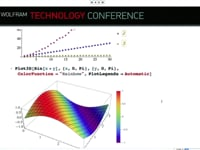Plot Legends22:49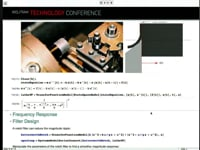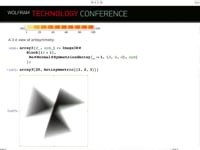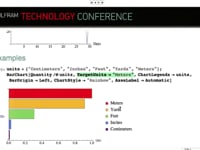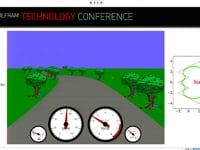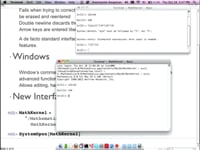Queueing Networks22:57